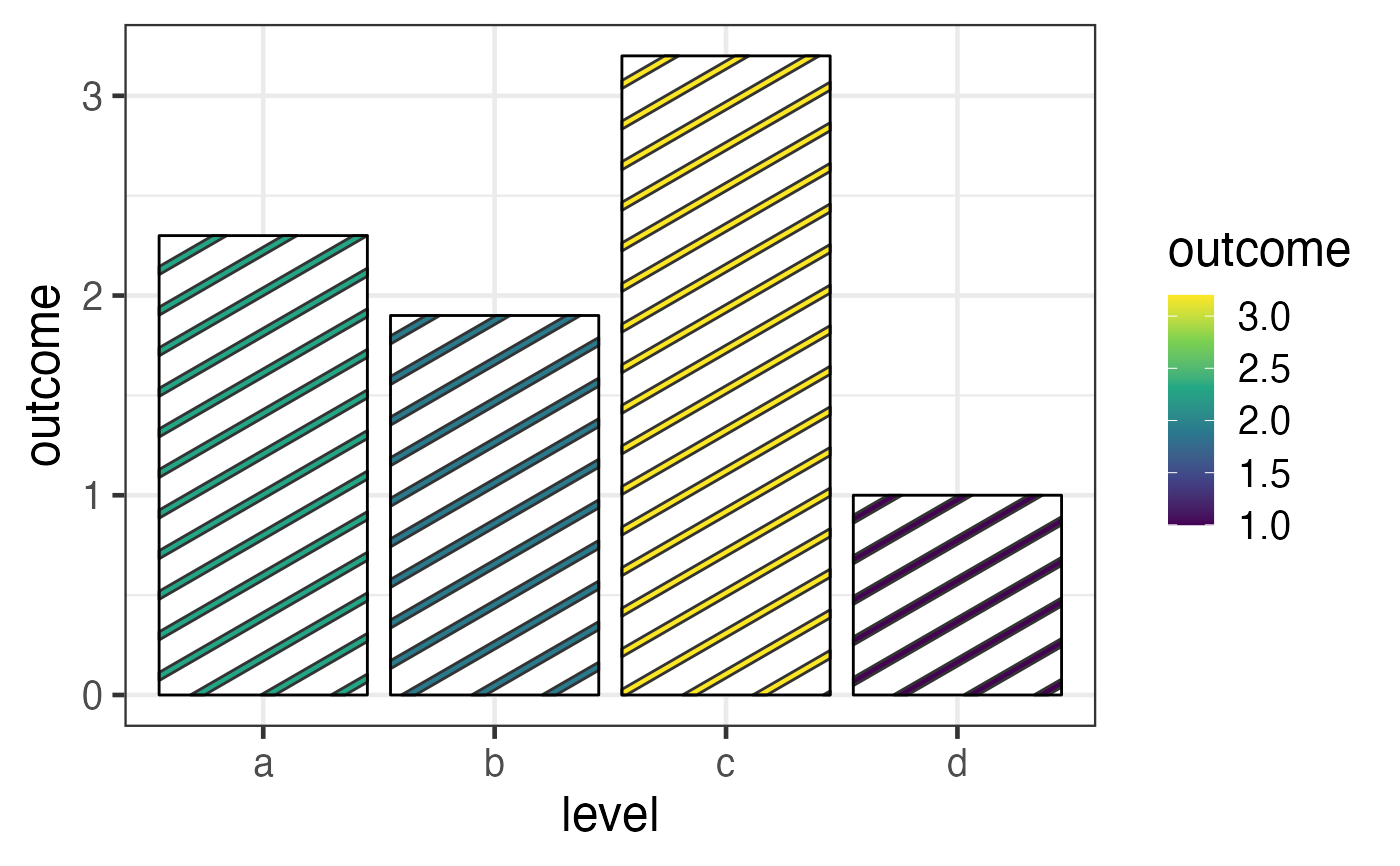The viridis scales provide colour maps that are perceptually uniform in both colour and black-and-white. They are also designed to be perceived by viewers with common forms of colour blindness. See also https://bids.github.io/colormap/.

scale_pattern_colour_viridis_d(
...,
alpha = 1,
begin = 0,
end = 1,
direction = 1,
option = "D",
aesthetics = "pattern_colour"
)

scale_pattern_fill_viridis_d(
...,
alpha = 1,
begin = 0,
end = 1,
direction = 1,
option = "D",
aesthetics = "pattern_fill"
)

scale_pattern_fill2_viridis_d(
...,
alpha = 1,
begin = 0,
end = 1,
direction = 1,
option = "D",
aesthetics = "pattern_fill2"
)

scale_pattern_colour_viridis_c(
...,
alpha = 1,
begin = 0,
end = 1,
direction = 1,
option = "D",
values = NULL,
space = "Lab",
na.value = "grey50",
guide = guide_colourbar(available_aes = "pattern_colour"),
aesthetics = "pattern_colour"
)

scale_pattern_fill_viridis_c(
...,
alpha = 1,
begin = 0,
end = 1,
direction = 1,
option = "D",
values = NULL,
space = "Lab",
na.value = "grey50",
guide = guide_colourbar(available_aes = "pattern_fill"),
aesthetics = "pattern_fill"
)

scale_pattern_fill2_viridis_c(
...,
alpha = 1,
begin = 0,
end = 1,
direction = 1,
option = "D",
values = NULL,
space = "Lab",
na.value = "grey50",
guide = guide_colourbar(available_aes = "pattern_fill2"),
aesthetics = "pattern_fill2"
)

Arguments

...

Other arguments passed on to discrete_scale(), continuous_scale(), or binned_scale to control name, limits, breaks, labels and so forth.

begin, end, alpha, direction, option, values, space, na.value, guide

aesthetics

Character string or vector of character strings listing the name(s) of the aesthetic(s) that this scale works with. This can be useful, for example, to apply colour settings to the colour and fill aesthetics at the same time, via aesthetics = c("colour", "fill").

Value

A ggplot2::Scale object.

Examples

if (require("ggplot2")) {
df <- data.frame(level = c("a", "b", "c", "d"),
outcome = c(2.3, 1.9, 3.2, 1))
# discrete 'viridis' palette
gg <- ggplot(df) +

aes(level, outcome, pattern_fill = level),
pattern = 'stripe',
fill    = 'white',
colour  = 'black'
) +
theme_bw(18) +
scale_pattern_fill_viridis_d()
plot(gg)

# continuous 'viridis' palette
gg <- ggplot(df) +

aes(level, outcome, pattern_fill = outcome),
pattern = 'stripe',
fill    = 'white',
colour  = 'black'
) +
theme_bw(18) +
scale_pattern_fill_viridis_c()
plot(gg)
}